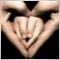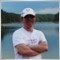# EA based on direction change of moving average35

I'm trying to create an EA based on change in direction of a moving average. Actually I have written the code, but it doesn't trade when I backtest it. It should create a BUY when the MA changes direction from negative to positive and a SELL when it changes from positive to negative. It should also close any existing SELL orders when it generates a buy and vice versa.

I have written others that have performed properly, but this is my first attempt at opening and closing orders based on the same indicator. Any help would be greatly appreciated.164435

DxdCn:
I don't understand what you're saying.16558

dlindsey:
DxdCn:
I don't understand what you're saying.124

dlindsey:

I'm trying to create an EA based on change in direction of a moving average. Actually I have written the code, but it doesn't trade when I backtest it. It should create a BUY when the MA changes direction from negative to positive and a SELL when it changes from positive to negative. It should also close any existing SELL orders when it generates a buy and vice versa.

I have written others that have performed properly, but this is my first attempt at opening and closing orders based on the same indicator. Any help would be greatly appreciated.

dlindsey.

thanks.35

evaluator:
dlindsey:

I'm trying to create an EA based on change in direction of a moving average. Actually I have written the code, but it doesn't trade when I backtest it. It should create a BUY when the MA changes direction from negative to positive and a SELL when it changes from positive to negative. It should also close any existing SELL orders when it generates a buy and vice versa.

I have written others that have performed properly, but this is my first attempt at opening and closing orders based on the same indicator. Any help would be greatly appreciated.

dlindsey.

thanks.
Files:639

Put Comment in your code and see why.
Comment(chgdirup, " ", lastdir, " ", EMA0, " ", EMA1);35

bonechair:
Put Comment in your code and see why.
Comment(chgdirup, " ", lastdir, " ", EMA0, " ", EMA1);
I'm surry, but I don't understand. I'm quite new at this and don't understand how that would help.639

```
EMA0 = iMA(NULL,0,EMA,0,MODE_EMA,PRICE_CLOSE,0);
EMA1 = iMA(NULL,0,EMA,0,MODE_EMA,PRICE_CLOSE,1);

//check for change of direction
if (EMA0>EMA1 && lastdir==0)
{
lastdir = 1;
}

if (EMA0<EMA1 && lastdir==0)
{
lastdir = 2;
}

if (EMA0>EMA1 && lastdir == 2)
{
chgdirup = 1;
lastdir = 1;
}

if (EMA0<EMA1 && lastdir == 1)
{
chgdirup = 2;
lastdir = 2;
}
Comment(chgdirup, " ", lastdir, " ", EMA0, " ", EMA1);
```
Im looking at this part and seeing that chgdirup stays 0 but lastdir keeps changing, dunno why man.

You must put int lastdir = 0; before the start() otherwise it resets to 0 everytime. I did this but still not work.639

There still some glitches in here but here you go:

```//+-------------------------------------+
//|                   gbp emachange.mq4 |
//|                          Don Lindsey|
//+-------------------------------------+

//This expert advisor determines a change of direction in a moving average and opens
//and closes trades accordingly. If the change in direction is from downward to
//upward a buy order is placed and, if a sell trade is active, closes it. If the
//change in direction is from upward to downward a sell order is placed and, if a
//buy order is active, closes it.
//This is accomplished with the integer variable "lastdir" and the boolean variable
//"chgdirup." "lastdir" tracks the moving average's direction and "changedir"
//indicates a change in direction has occurred. When "chgdirup" goes true, open sell
//orders are closed and a buy order is placed. When "chgdirup" becomes false, open
//buy orders are closed and a sell order is placed.

#include <stdlib.mqh>

extern int expertId = 1150;

extern double StopLoss = 50;
extern double TakeProfit = 500;
extern double Lots = 0.1;
extern double TrailingStop = 0;
extern int EMA = 15;

extern int    slippage=2;   	//slippage for market order processing
extern int    shift=1;			//shift to current bar,

extern int    OrderTriesNumber=5; //to repeate sending orders when got some error

extern string    EAName="gbp emachange";

//extern int TimeStart = 800;
//extern int TimeStop = 1900;

extern double MinLot = 0.1;    //minimum lot size
extern double MaxLot = 200;    //maximum lot size

extern bool   FixedLot = false; //trigger to use variable lot size
bool chgdirup;
int lastdir = 0;
int start()
{

int cnt, ticket, total;
double EMA0, EMA1;

//bool changedirup = false;
//bool changedirdown = false;

// initial data checks
// it is important to make sure that the expert works with a normal chart and the user did not make any //mistakes setting external variables (Lots, StopLoss, TakeProfit, TrailingStop) in our case, we check //TakeProfit on a chart of less than 100 bars
if(Bars<100)
{
Print("bars less than 100");
return(0);
}
if(TakeProfit<10)
{
Print("TakeProfit less than 10");
return(0);  // check TakeProfit
}

total = OrdersTotal();
if(total<1)
{
// no opened orders identified
if(AccountFreeMargin()<(1000*Lots))
{
Print("We have no money. Free Margin = ", AccountFreeMargin());
return(0);
}
//double LotsOptimized()

double lot=Lots;

//---- select lot size
lot=NormalizeDouble(AccountFreeMargin()/(StopLoss*200),1);
if (lot<0.1) Lots=0.1;
if (lot>200) Lots=200;
else Lots=lot;

// to simplify the coding and speed up access, data are put into internal variables

EMA0 = iMA(NULL,0,EMA,0,MODE_EMA,PRICE_CLOSE,0);
EMA1 = iMA(NULL,0,EMA,0,MODE_EMA,PRICE_CLOSE,1);

//check for change of direction
if (EMA0>EMA1 && lastdir==0)
{
lastdir = 1;
}

else

if (EMA0<EMA1 && lastdir==0)
{
lastdir = -1;
}

else

if (EMA0>EMA1 && lastdir == -1)
{
chgdirup = true;
lastdir = 1;
}

else

if (EMA0<EMA1 && lastdir == 1)
{
chgdirup = false;
lastdir = -1;
}

//    int ct=Hour()*100+Minute();
// time restrictions for entry
// if (( ct<TimeStart) || ( ct>TimeStop))
// {
//        return;
//  }

// check for long position (BUY) possibility
if (chgdirup == true)
{
if (OrderType() == (OP_SELL))
{
OrderClose(OrderTicket(),OrderLots(),Bid,3,Violet); // close position
}
}
else
{
return(0);
}

if(ticket>0)
{
}
else
{
Print("Error opening BUY order : ",GetLastError());
return(0);
}

// check for short position (SELL) possibility
if(chgdirup == false)
{
ticket=OrderSend(Symbol(),OP_SELL,Lots,Bid,3,Bid+StopLoss*Point,Bid-TakeProfit*Point,"gbp emachange",16384,0,Red);
{
}
}
else
{
return(0);
}

if(ticket>0)
{
if(OrderSelect(ticket,SELECT_BY_TICKET,MODE_TRADES)) Print("SELL order opened : ",OrderOpenPrice());
}
else {
Print("Error opening SELL order : ",GetLastError());
return(0);
}

// it is important to enter the market correctly, but it is more important to exit it correctly...

//   for(cnt=0;cnt<total;cnt++)
//     {
//      if(OrderType()<=OP_SELL &&   // check for opened position
//         OrderSymbol()==Symbol())  // check for symbol
//        {
//         if(OrderType()==OP_BUY)   // long position is opened
//           {
// should it be closed?
//      if(chgdirup==false)
//              {
//             OrderClose(OrderTicket(),OrderLots(),Bid,3,Violet); // close position
//            return(0); // exit
//                }
//         check for trailing stop
//            if(TrailingStop>0)
//              {
//               if(Bid-OrderOpenPrice()>Point*TrailingStop)
//                {
//                 if(OrderStopLoss()<Bid-Point*TrailingStop)
//                    {
//     OrderModify(OrderTicket(),OrderOpenPrice(),Bid-*TrailingStop,OrderTakeProfit(), 0,Green);
//                     return(0);
//                 }
//                 }
//              }
//          }
//       else // go to short position
//               {
//               Print ("Something needs to be here.");
//               }
// should it be closed?
//   if(chgdirup==false)
//             {
//              return(0); // exit
//             }
// check for trailing stop
//            if(TrailingStop>0)
//              {
//                {
//                    {
//                     return(0);
//                    }
//                }
//              }

}
return(0);
}

// end of program.

```
You had a return; in the middle there that was stopping the whole code.639

else
{
return(0);
}

This should come out.

Not bad on the 4 Hour with 10 pip takeprofit, some months up, some months down(mostly down).